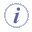# Copy/Pasting Parameters

The Tools>Options>General>Parameters and Measure check boxes allow you to:

 Paste a parameter without the formula which defines it. For example: `Holeplus= 15 = Diameter + 10````  will be pasted as Real.i = 15   ``` (if the With Value box is selected) Paste a parameter as well as the formula which defines it, but only if the parameters referred to in the formula are also selected in the copy. For example: `Holeplus= 15 = Diameter + 10```` will be pasted as Real.i = 15 ``` if the Diameter parameter does not belong to the items selected for the copy but HolePlus will be pasted as `Real.i = 15 = Real.j + 10` if Diameter is selected in the copy (use multiselection). Paste a parameter as well as the formula. `Holeplus= 15 = Diameter + 10```` will be pasted as ````Real.i = Diameter + 10`When copying parameters sets containing hidden parameters, these parameters are automatically pasted when pasting the parameters sets and appear as hidden parameters.